# VS2.2 - Illustration of PCDs in One Interval

#### 2023-04-28

First we load the pcds package:

library(pcds)

# 1 Functions for PCDs with Vertices in One Interval

For illustration of construction of PCDs and related quantities, we first focus on one Delaunay cell, which is an interval in the 1D setting. Due to scale invariance of PE- and CS-PCDs with vertices from uniform distribution in a bounded interval in $$\mathbb R$$, most data generation and computations can be done in the unit interval $$[0,1]$$. Here, we do not treat AS-PCDs separately, as it is a special case of PE-PCDs with expansion parameter $$r=2$$. Also, geometry invariance and scale invariance for uniform data on bounded intervals in 1D space are equivalent.

Most of the PCD functions we will illustrate in this section are counterparts of the functions in the multiple-interval case in Section “VS1_3_1DArtiData” for an arbitrary interval and sometimes for the unit interval $$(0,1)$$ (for speed and ease of computation).

For more detail on the construction of PCDs in the 1D setting, see Ceyhan (2012), Ceyhan (2016) and Ceyhan (2020).

c<-.4
a<-0; b<-10; int<-c(a,b)
n<-5 #try also n=10, 50, 100

We first choose an arbitrary interval $$[a,b]$$ with vertices $$a=$$ 0 and $$b=$$ 10, and generate $$n=$$ 5 $$\mathcal{X}$$ points inside this interval using the function runif in base R, and to construct the vertex regions choose the centrality parameter $$c=$$ 0.4 which corresponds to $$M_c=$$ 4 in this interval. $$\mathcal{X}$$ points are denoted as Xp and $$\mathcal{Y}$$ points in Section “VS1_3_1DArtiData” correspond to the vertices $$\{a,b\}$$ (i.e., end points of the interval $$[a,b]$$.

xf<-(int-int)*.1

set.seed(123)
Xp<-runif(n,a-xf,b+xf)

Plot of the interval $$[a,b]$$ and the $$\mathcal{X}$$ points in it.

Xp2 =c(Xp,int)
Xlim<-range(Xp2)
Ylim<-.005*c(-1,1)
xd<-Xlim-Xlim
plot(Xp2,rep(0,n+2),xlab="x", ylab=" ",xlim=Xlim+xd*c(-.05,.05), yaxt='n',
ylim=Ylim,pch=".",cex=3,main="X Points with One Interval")
abline(h=0,lty=2)
#now, we add the intervals based on Y points
par(new=TRUE)
plotIntervals(Xp,int,xlab="",ylab="",main="")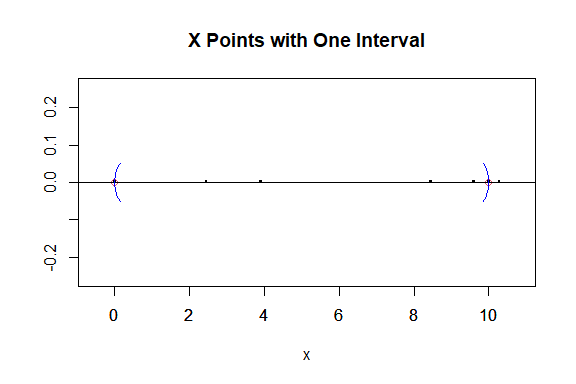Figure 1.1: Scatterplot of the uniform $$X$$ points in the interval $$(0,10)$$.

Alternatively, we can use the plotIntervals function in pcds to obtain the same plot by executing plotIntervals(Xp,int,xlab="",ylab="") command.

## 1.1 Functions for Proportional Edge PCDs with Vertices in One Interval

We use the same interval int and centrality parameter c= 0.4 and the generated data above.

And we choose the expansion parameter $$r=$$ 1.5 to illustrate the PE proximity region construction.

The function NPEint is used for the construction of PE proximity regions taking the arguments x,int,r,c where

• x, a 1D point for which PE proximity region is constructed,
• r, a positive real number which serves as the expansion parameter in PE proximity region; must be $$\ge 1$$,
• c, a positive real number in $$(0,1)$$ parameterizing the center inside int$$=(a,b)$$ with the default c=.5, For the interval, int$$=(a,b)$$, the parameterized center is $$M_c=a+c(b-a)$$.
• int, a vector of two real numbers representing an interval.

NPEint returns the proximity region as the end points of the interval proximity region.

r<-1.5
NPEint(7,int,r,c)
#>   5.5 10.0
NPEint(Xp,int,r,c)
#>  0.000000 3.676395

Indicator for the presence of an arc from a (data or $$\mathcal{X}$$) point to another for PE-PCDs is the function IndNPEint which takes arguments p1,p2,int,r,c where

• p1 a 1D point whose PE proximity region is constructed,
• p2 another 1D point. The function determines whether p2 is inside the PE proximity region of p1 or not, and
• int,r,c are as in NPEint.

This function returns $$I(x_2 \in N_{PE}(x_1,r,c))$$ for $$x_2$$, that is, returns 1 if $$x_2$$ in $$N_{PE}(x_1,r,c)$$, 0 otherwise. One can use it for points in the data set or for arbitrary points (as if they were in the data set).

IndNPEint(7,7,int,r,c)
#>  1
IndNPEint(Xp,Xp,int,r,c)
#>  0

Number of arcs of the PE-PCD can be computed by the function NumArcsPEint with arguments Xp,int,r,c where

• Xp is a set of 1D points which constitute the vertices of PE-PCD
• int,r,c are as in NPEint.

The output is as in NumArcsPE1D.

NumArcsPEint(Xp,int,r,c)
#> $num.arcs #>  2 #> #>$num.in.range
#>  4
#>
#> $num.in.intervals #>  0 4 1 #> #>$int.num.arcs
#>  0 2 0
#>
#> $data.interval.indices #>  2 2 2 2 3 The incidence matrix of the PE-PCD for the one interval case can be found by IncMatPEint, using the IncMatPEint(Xp,int,r,c) command. It has the same arguments as NumArcsPEint function. Plot of the arcs in the digraph PE-PCD with vertices in or outside one interval is provided by the function plotPEarcs.int which has the arguments Xp,int,r,c=.5,Jit=.1,main=NULL,xlab=NULL,ylab=NULL,xlim=NULL,ylim=NULL,center=FALSE, ... where int is a vector of two 1D points constituting the end points of the interval and the other arguments are as in plotPEarcs1D. The digraph is based on the $$M_c$$-vertex regions with $$M_c=$$ 4. Arcs are jittered along the $$y$$-axis to avoid clutter on the real line for better visualization. Plot of the arcs of the above PE-PCD, together with the center (with the option center=TRUE) is provided below. jit<-.1 set.seed(1) plotPEarcs.int(Xp,int,r=1.5,c=.3,jit,xlab="",ylab="",center=TRUE)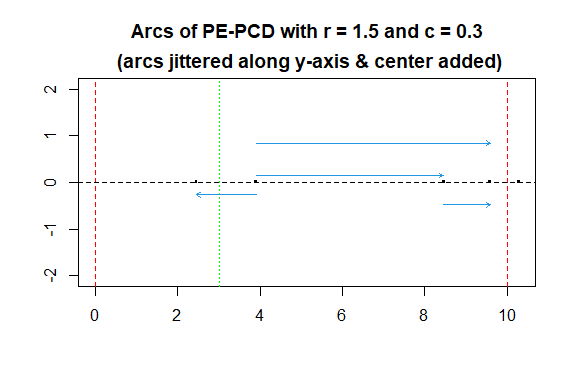Figure 1.2: The arcs of the PE-PCD for a 1D data set, the end points of the interval (red) and the center (green) are plotted with vertical dashed lines. Plots of the PE proximity regions (or intervals) in the one-interval case is provided with the function plotPEarcs.int. The proximity regions are plotted with the center with center=TRUE option. set.seed(1) plotPEregs.int(Xp,int,r,c,xlab="x",ylab="",center = TRUE)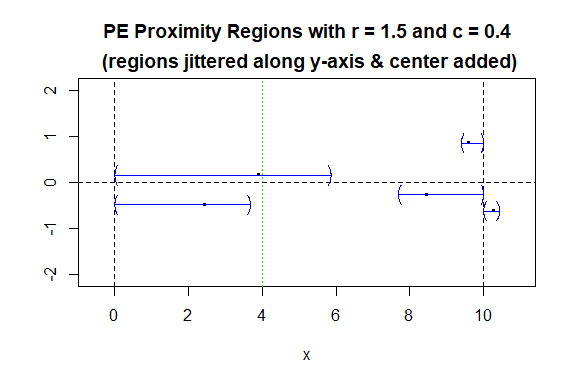Figure 1.3: The PE proximity regions for 10 $$X$$ points on the real line, the end points of the interval (black) and the center (green) are plotted with vertical dashed lines. The function ArcsPEint is an object of class “PCDs” with arguments are as in NumArcsPEint and the output list is as in the function ArcsPE1D. The plot function returns the same plot as in plotPEarcs.int, hence we comment it out below. Arcs<-ArcsPEint(Xp,int,r,c) Arcs #> Call: #> ArcsPEint(Xp = Xp, int = int, r = r, c = c) #> #> Type: #>  "Proportional Edge Proximity Catch Digraph (PE-PCD) for 1D Points with Expansion Parameter r = 1.5 and Centrality Parameter c = 0.4" summary(Arcs) #> Call: #> ArcsPEint(Xp = Xp, int = int, r = r, c = c) #> #> Type of the digraph: #>  "Proportional Edge Proximity Catch Digraph (PE-PCD) for 1D Points with Expansion Parameter r = 1.5 and Centrality Parameter c = 0.4" #> #> Vertices of the digraph = Xp #> Partition points of the region = int #> #> Selected tail (or source) points of the arcs in the digraph #> (first 6 or fewer are printed) #>  8.459662 3.907723 #> #> Selected head (or end) points of the arcs in the digraph #> (first 6 or fewer are printed) #>  9.596209 2.450930 #> #> Parameters of the digraph #>$centrality parameter
#>  0.4
#>
#> $expansion parameter #>  1.5 #> #> Various quantities of the digraph #> number of vertices number of partition points #> 5.0 2.0 #> number of intervals number of arcs #> 1.0 2.0 #> arc density #> 0.1 plot(Arcs) ## 1.2 Functions for Construction and Simulation of Central Similarity PCDs with Vertices in One Interval We use the same interval int and centrality parameter c=.4 and the generated data above, and choose the expansion parameter $$\tau=$$ 1.5 to illustrate the CS proximity region construction. The function NCSint is used for the construction of CS proximity regions taking the same arguments as NPEint with expansion parameter r being replace by t (which must be positive). In fact, most functions for CS PCDs in the 1D setting admit the same arguments as their counterparts for PE PCDs, with expansion parameter r being replace by t. NCSint returns the proximity region as the end points of the interval proximity region. tau<-1.5 NCSint(Xp,int,tau,c) #>  0 10 Indicator for the presence of an arc from a (data or $$\mathcal{X}$$) point to another for CS-PCDs is the function IndNCSint which takes the same arguments as IndNPEint with expansion parameter r replaced with t. This function returns $$I(x_2 \in N_{PE}(x_1,r,c))$$ for $$x_2$$, that is, returns 1 if $$x_2$$ in $$N_{PE}(x_1,r,c)$$, 0 otherwise. One can use it for points in the data set or for arbitrary points (as if they were in the data set). IndNCSint(Xp,Xp,int,tau,c) #try also IndNCSint(Xp,Xp,int,tau,c) #>  0 Number of arcs of the CS-PCD can be computed by the function NumArcsCSint which has the same arguments as NumArcsPEint with expansion parameter r replaced with t. The output of this function is as in NumArcsCS1D. NumArcsCSint(Xp,int,tau,c) #>$num.arcs
#>  5
#>
#> $num.in.range #>  4 #> #>$num.in.ints
#>  0 4 1
#>
#> $int.num.arcs #>  0 5 0 #> #>$data.int.ind
#>  2 2 2 2 3

The incidence matrix of the CS-PCD for the one interval case can be found by IncMatCSint, using the IncMatCSint(Xp,int,tau,c) command. It has the same arguments as NumArcsCSint function.

Plot of the arcs in the digraph CS-PCD with vertices in or outside one interval is provided by the function plotCSarcs.int which has the same arguments as plotPEarcs.int with expansion parameter r replaced with t. The digraph is based on the $$M_c$$-vertex regions with $$M_c$$=4. Arcs are jittered along the $$y$$-axis to avoid clutter on the real line for better visualization.

Plot of the arcs of the above CS-PCD, together with the center (with the option center=TRUE) is provided below.

set.seed(1)
plotCSarcs.int(Xp,int,t=1.5,c=.3,jit,xlab="",ylab="",center=TRUE)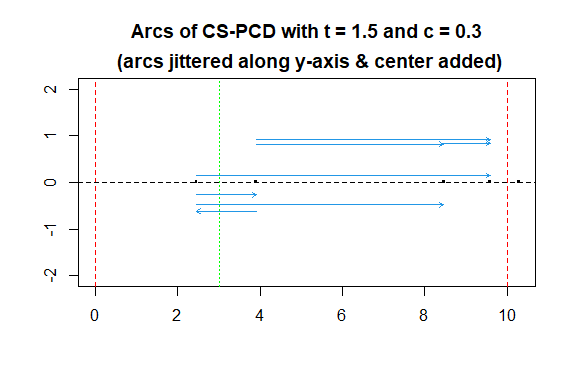Figure 1.4: The arcs of the CS-PCD for a 1D data set, the end points of the interval (red) and the center (green) are plotted with vertical dashed lines.

Plots of the CS proximity regions (or intervals) in the one-interval case is provided with the function plotCSarcs.int. The proximity regions are plotted with the center with center=TRUE option.

set.seed(1)
plotCSregs.int(Xp,int,tau,c,xlab="x",ylab="",center=TRUE)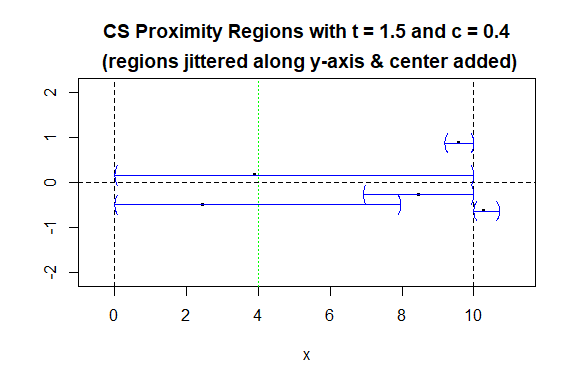Figure 1.5: The CS proximity regions for 10 $$X$$ points on the real line, the end points of the interval (black) and the center (green) are plotted with vertical dashed lines.

The function ArcsPEint is an object of class “PCDs” with arguments are as in NumArcsCSint and the output list is as in the function ArcsCS1D. The plot function returns the same plot as in plotCSarcs.int, hence we comment it out below.

Arcs<-ArcsCSint(Xp,int,tau,c)
Arcs
#> Call:
#> ArcsCSint(Xp = Xp, int = int, t = tau, c = c)
#>
#> Type:
#>  "Central Similarity Proximity Catch Digraph (CS-PCD) for 1D Points with Expansion Parameter t = 1.5 and Centrality Parameter c = 0.4"
summary(Arcs)
#> Call:
#> ArcsCSint(Xp = Xp, int = int, t = tau, c = c)
#>
#> Type of the digraph:
#>  "Central Similarity Proximity Catch Digraph (CS-PCD) for 1D Points with Expansion Parameter t = 1.5 and Centrality Parameter c = 0.4"
#>
#>  Vertices of the digraph =  Xp
#>  Partition points of the region =  int
#>
#>  Selected tail (or source) points of the arcs in the digraph
#>       (first 6 or fewer are printed)
#>  2.450930 8.459662 3.907723 3.907723 3.907723
#>
#>  Selected head (or end) points of the arcs in the digraph
#>       (first 6 or fewer are printed)
#>  3.907723 9.596209 2.450930 8.459662 9.596209
#>
#> Parameters of the digraph
#> $centrality parameter #>  0.4 #> #>$expansion parameter
#>  1.5
#>
#> Various quantities of the digraph
#>         number of vertices number of partition points
#>                       5.00                       2.00
#>        number of intervals             number of arcs
#>                       1.00                       5.00
#>                arc density
#>                       0.25
plot(Arcs)

## 1.3 Auxiliary Functions to Define Proximity Regions for Points in an Interval

PCDs in the 1D setting are constructed using the vertex regions based on a center or central point inside the support interval(s). We illustrate a center or central point in an interval. The function centMc takes arguments int,c and returns the central point $$M_c=a+c(b-a)$$ in the interval $$[a,b]$$. On the other hand, the function centersMc takes arguments Yp,c where Yp is a vector real numbers that constitute the end points of intervals. It returns the central points of intervals based on the order statistics of a set 1D points Yp.

c<-.4 #try also c<-runif(1)
a<-0; b<-10
int = c(a,b)
centMc(int,c)
#>  4

n<-5 #try also n=10, 50, 100
y<-runif(n)
centersMc(y,c)
#>  0.2887174 0.6417875 0.7558345 0.9169039

The function rv.mid.int takes arguments p,int,c where

• p, a 1D point for which the vertex region is to be found and
• int,c are as in centMc. This function returns the index of the vertex region in a middle interval that contains a given point.
c<-.4
a<-0; b<-10; int = c(a,b)
rv.mid.int(6,int,c)
#> $rv #>  2 #> #>$int
#> vertex 1 vertex 2
#>        0       10

We illustrate the vertex regions using the following code. We annotate the vertices with corresponding indices with rv=i for $$i=1,2$$, and also generate $$n=$$ 5 points inside the interval $[$0 , 10 $$]$$ and provide the scatterplot of these points (jittered along the $$y$$-axis for better visualization) labeled according to the vertex region they reside in. Type also ? rv.mid.int for the code to generate this figure.

The function rv.end.int takes arguments p,int for the point whose vertex region is to be found and the support interval. It returns the index of the end interval that contains a given point, 1 for left end interval 2 for right end interval.

a<-0; b<-10; int<-c(a,b)
rv.end.int(-6,int)
#> $rv #>  1 #> #>$int
#> vertex 1 vertex 2
#>        0       10
rv.end.int(16,int)
#> $rv #>  2 #> #>$int
#> vertex 1 vertex 2
#>        0       10